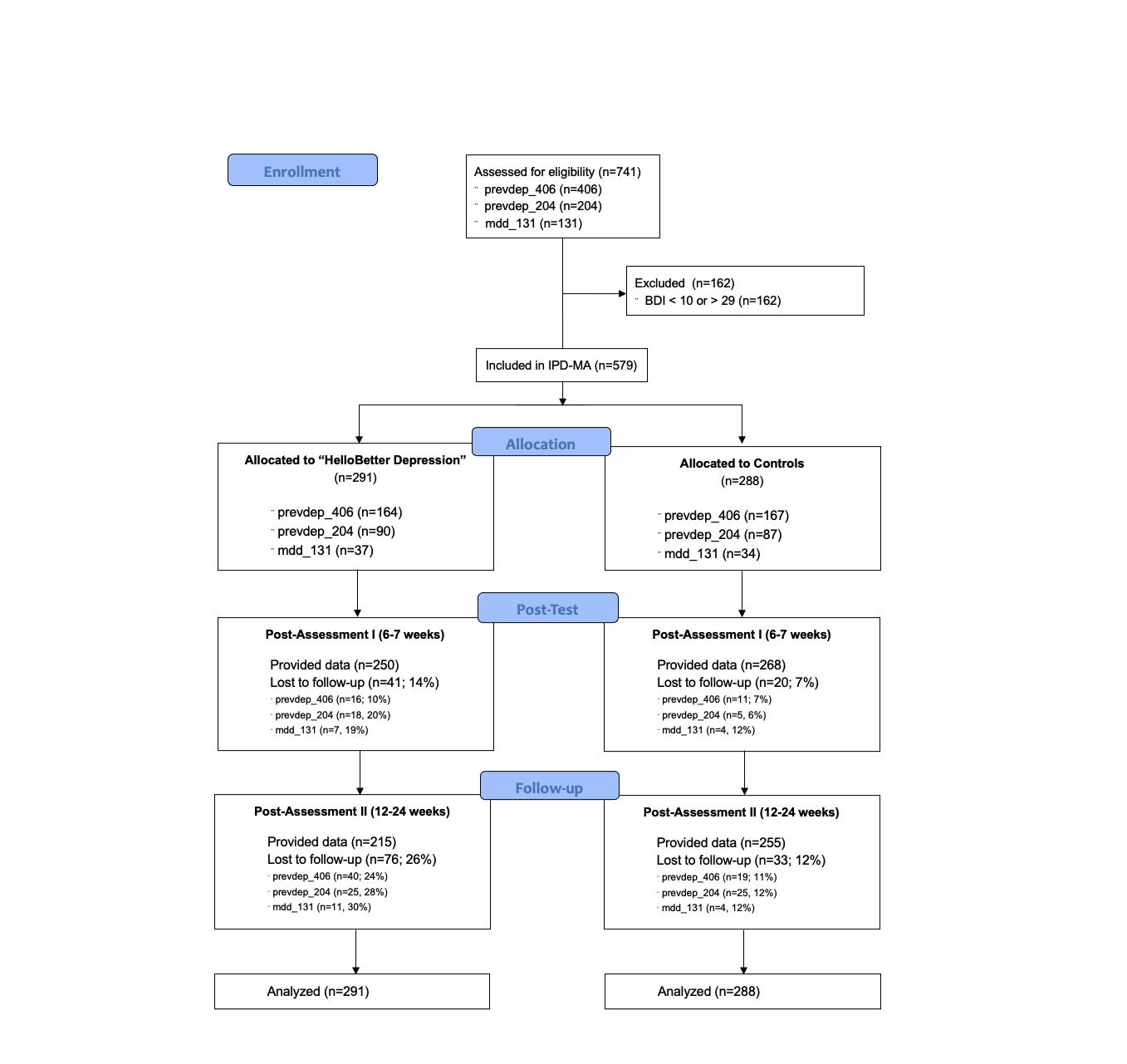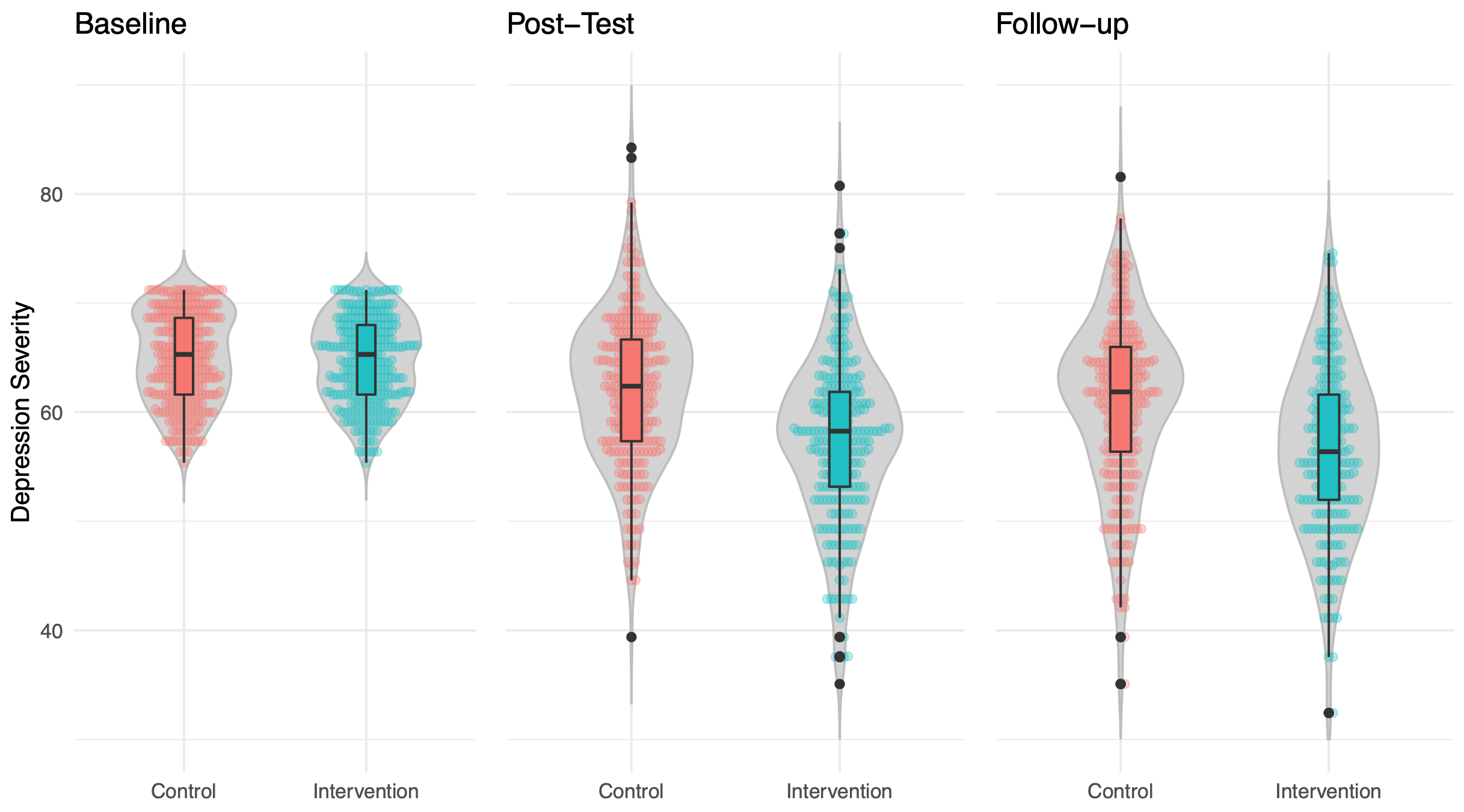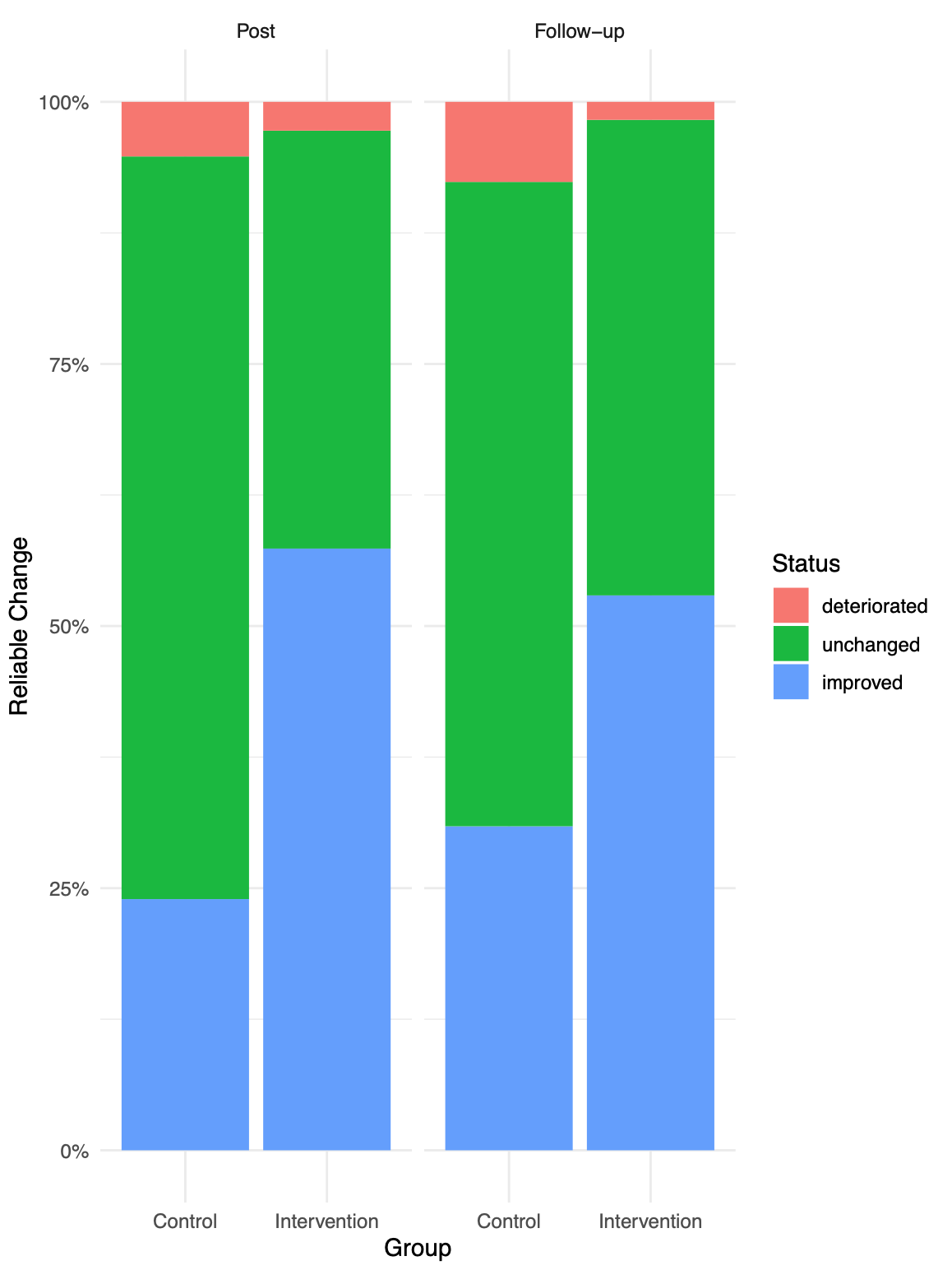1FAU Erlangen-Nürnberg, 2Get.On Institut für Gesundheitstrainings GmbH/HelloBetter

Access Analysis Repository ↗

# Abstract

Aims: This individual patient data (IPD) meta-analysis aims to evaluate the overall effectiveness of an Internet- and mobile-based Intervention (“HelloBetter Depression”) in individuals with mild to moderate depression.

Methods: We included individual patient data of $$k=3$$ randomized controlled studies examining the effects of “HelloBetter Depression” compared to control groups receiving psychoeducation ($$N_{total} = 579$$). Only patients with mild to moderate depressive symptoms (BDI 10-29) were included. One-step IPD meta-analysis procedures were used to obtain pooled effect estimates. Primary outcome was the depressive symptom severity at post-test (6-7 weeks). Secondary outcomes included results on depression at 12-24 week follow-up, as well as effects on anxiety symptoms and behavioral activation.

Results: At post-test, a significant within-group ($$d$$=0.74; 95%CI: 0.64-0.85) and between-group effect ($$d$$=0.46; 95%CI: 0.25-0.66) favoring the intervention was found. Significantly more individuals in the intervention groups ($$n$$=167, 57.38%) achieved reliable response at post-test compared to controls ($$n$$=69, 23.95%). Effects were sustained at follow-up. We also found significant effects on anxiety symptoms ($$d_{within}$$=0.62-0.64; $$d_{between}$$=0.34-0.37) and behavioral activation ($$d_{within}$$=0.80-0.90; $$d_{between}$$=0.33-0.54) at both post-test and follow-up. No negative intervention effects on depressive symptoms could be detected.

Discussion: Results of this IPD meta-analysis indicate that “HelloBetter Depression” can effectively reduce symptoms of depression and anxiety, as well as increase behavioral activation in patients with mild to moderate depressive symptoms.

Trial Registration: DRKS00004709, DRKS00005973, DRKS00005025.

# 1 Aims

In this individual patient data (IPD) meta-analysis, we aim to evaluate the effectiveness of an Internet- and mobile-based intervention for depression (“HelloBetter Depression”). We hypothesized the intervention to be superior to control groups in terms of effects on depressive symptoms, as well as with respect to the proportion of individuals achieving reliable response.

# 2 Method

The trials investigated in this study have been registered in the German clinical trials register (DRKS00004709, DRKS00005973, DRKS00005025). We present the methods and results of this secondary analysis in accordance to the CONSORT Statement (Moher et al., 2010), and the Guidelines for Executing and Reporting Research on Internet Interventions (Proudfoot et al., 2011). The Preferred Reporting Items for Systematic Reviews and Meta-Analyses (PRISMA; Moher et al., 2009) are followed only were applicable, since data used in this study is not based on a comprehensive literature search. The material used for the analyses in this study has been made openly available in an Open Science Framework (OSF) repository.

A total of $$k=3$$ primary studies were included in this IPD meta-analysis. These trials will be referenced as prevdep_406, prevdep_204 and mdd_131 throughout the report. Included studies are previously conducted randomized controlled trials evaluating the intervention “HelloBetter Depression” (research title: “Get.On Mood Enhancer”). Detailed reports of these primary studies have been published elsewhere in peer-reviewed publications (prevdep_406: Buntrock et al., 2015; prevdep_204: Ebert et al., 2018; mdd_131: Reins et al., 2019).

## 2.1 Design

The included studies are two-armed randomized controlled trials. The intervention groups (IG; prevdep_406: $$n$$=164; prevdep_204: $$n$$=90; mdd_131: $$n$$ = 37; $$n_{total}$$ = 291) received “HelloBetter Depression”, and Internet- and mobile-based depression intervention. Control groups (CG; prevdep_406: $$n$$=167; prevdep_204: $$n$$=87; mdd_131: $$n$$ = 34; $$n_{total}$$ = 288) received brief psychoeducation for depressive symptoms. Assuming a fixed-effect inverse-variance pooling model, this sample size allows for detecting an effect of $$SMD = 0.24$$ while maintaining a sufficient power of $$1-\beta = 0.8$$ (Harrer et al., 2019).

Participants in all primary studies were assessed at baseline (T0), post-treatment (T1; 6-7 weeks after baseline), and follow-up (T2; prevdep_406 and prevdep_204: 24 weeks; mdd_131: 12 weeks after baseline).

## 2.2 Participants

Primary study prevdep_406 originally included $$N$$=406 participants from the general population recruited via a large statutory health insurance company. Included participants were adults with subthreshold depression (ICD-10 equivalent F32.0; Centre for Epidemiological Studies’ Depression Scale score ≥ 16, no current Major Depression according to Diagnostic and Statistical Manual of Mental Disorders criteria).

Study prevdep_204 originally included $$N$$=204 participants recruited in the general population via a large health insurance. For inclusion, participants had to suffer from subthreshold depression (ICD-10 equivalent F32.0; Centre for Epidemiological Studies’ Depression Scale score ≥ 16, no current Major Depression according to Diagnostic and Statistical Manual of Mental Disorders criteria) at baseline.

Study mdd_131 originally included $$N$$=131 participants, which were also recruited from the general population through a large health insurance. To be eligible for inclusion, participants had to suffer from major depression (ICD-10 equivalent F32.1 and F32.2; current Major Depression according to Diagnostic and Statistical Manual of Mental Disorders criteria) at baseline.

Further eligibility criteria applied in all included primary studies were (i) being at least 18 years old, (ii) having Internet access, (iii) declaring willingness to provide self-report data at all three assessment points, and (iv) provision of informed consent.

To eligible for the present meta-analysis, participants had to experience mild to moderate symptoms of depression at baseline, as indicated by scores of 10-29 at baseline on the Beck Depression Inventory (BDI-I; Beck et al., 1961). These cut-off scores are based on the German S3 Leitlinie Unipolare Depression consensus statement (p. 177). This range of scores primarily covers symptom severities representative of ICD codes F32.0 and F32.1. Participants included in the primary studies with values outside the BDI-I 20-29 range were excluded from the meta-analysis.

## 2.3 Randomization

Randomization in all primary studies was conducted using an automated computer-based random integer generator (Randlist, Datinf GmbH, Tübingen, Germany). Randomization was conducted by a researcher who was not otherwise involved in the study. During the randomization procedure, allocation was concealed from participants, recruitment staff, diagnosticians, and e-coaches.

## 2.4 Intervention

All three studies evaluated “HelloBetter Depression” (Ebert et al., 2014); a guided self-help iCBT intervention consisting of six interactive sessions. Each session lasts about 45-60 minutes, though the duration might vary between users. The program was also available beyond the post assessment at 6-7 weeks. The modules rely on evidence-based face-to-face manuals that have been shown to be effective at reducing depressive symptomatology, including psychoeducation, and exercises for behavioral activation, problem solving, and relapse prevention. A strong emphasis was placed on homework assignments designed to integrate acquired coping skills into daily life. Participants were supported by eCoaches (psychotherapists-in-training supervised by an experienced clinician). Guidance took place in the form of individualized written feedback after each module. A motivational, adherence-focused feedback concept was employed.

## 2.5 Control Group

Participants in the control condition got access to a web-based psychoeducational intervention and care-as-usual. Psycho-educational interventions have been shown to be effective in reducing depressive symptoms and might serve as initial interventions in primary care (Donker et al., 2009). The psycho-educational intervention was based on the German S3-Guideline/National Disease Management Guideline for Unipolar Depression. It informed participants about the nature and evidence-based treatments of depression, including information about symptoms and sources of help. Offering the web-based psycho-educational intervention mimicked and enhanced usual care as information that patients might not always receive from their GP was systematically offered. Participants could go through the material as often as they wanted to. The psycho-educational intervention did not require participants to do homework assignments and there was no guidance.

## 2.6 Primary Outcome

The primary outcome were symptoms of depression at post-test (6-7 weeks after baseline). Instruments to assess depressive symptom severity differed in the primary studies. Studies prevdep_406 and prevdep_204 used the German version of the Center for Epidemiological Studies’ Depression Scale (CES-D) 20-item version (ADS; Hautzinger, Bailer, Hofmeister, & Keller, 2012; 20 items; range 0-60). Study mdd_131 operationalized depressive symptoms through the Patient Health Questionnaire 9 (PHQ-9; 9 items; range 0-27). Depressive symptom scores were therefore transformed to common metrics in order to allow joint analyses. A common metric is an Item Response Theory model, such as the GRM (Graded Response Model) or the GPCM (Generalized Partial Credit Model), that comprises parameters of items from various measures, measuring a common variable. Item parameters describe the relation between item response and latent variable. With such statistical model, one can estimate this common variable by subsets of items, e.g. if different measures are used or if data are missing. We used the common metrics model developed in Wahl et al. (2014). Common metrics were also used to determine BDI-I-based mild to moderate symptom scores, since this measure was not consistently used in the primary studies.

## 2.7 Secondary Outcomes

Secondary outcomes included anxiety as measured by the anxiety sub-scale of the Hospital Anxiety and Depression Scale (HADS-A; Zigmond & Snaith, 1983; 7 items; range 0-21), and behavioral activation as measured by the short form of the Behavioral Activation for Depression Scale (BADS-SF; Fuhr et al. 2016; 9 items; range 0-54). Secondary outcomes were measured at baseline, post-test and follow-up in all included primary studies. Client satisfaction with the intervention was assessed using the Client Satisfaction Questionnaire (adapted to the online context; CSQ-8; Boß et al., 2016; Nguyen, Attkisson, & Stegner, 1983; 8 items; IGs only).

## 2.8 Statistical Analyses

To evaluate the effectiveness of the intervention compared to the CG, analyses based on the intention-to-treat (ITT) principle were conducted. Analyses were conducted with R version 3.5.2 (R Core Team, 2013).

A joint modeling, multilevel-multiple imputation by chained equations (MICE) model was used to impute missing data (Jolani et al., 2015; Schafer & Yucel, 2002). Trial membership was used as a level-2 variable in the imputation model to account for the nested data structure (patients-in-trials). All subsequent analyses were conducted in the $$m$$=50 multiply imputed data sets. Test statistics and parameter estimates were calculated using Rubin’s rule (Barnard & Rubin, 1999).

We tested if the intervention was superior the active control in terms of (i) effects on participants’ depressive symptom severity and secondary outcomes from baseline to post-test (T1), and from baseline to three-month follow-up (T2). We also compared the proportion of participants with (ii) reliable response and (iii) reliable symptom deterioration between the IG and CG at T1 and T2. A significance level of 0.05 (two-sided) was used for all analyses.

Differences in effects between the two study conditions across the included primary studies were assessed using one-step IPD meta-analysis methods. We used linear mixed-effects models which included (1) a random study intercept and random group slope, as well as (2) a fixed-effect term controlling for baseline symptom severity to determine the overall intervention effects. To calculate effect sizes on depression (i.e. Cohen’s $$d$$), un-standardized group coefficients estimated in the linear mixed-effects models were divided by 10, exploiting that the common depression metric is standardized to have a population standard deviation of $$\sigma = 10$$. The pooled sample standard deviation at the assessed time point was used to standardize effects on secondary outcomes.

To determine if the depressive symptoms of patients had reliably decreased, we coded participants as responders or non-reponders using the Reliable Change Index (RCI; Jacobson & Truax, 1991). We compared the proportions of reliable responders in the IGs and CGs at post-test and follow-up using $$\chi^2$$-tests. Using the RCI, we also determined potential negative effects, defined as cases with a reliable depressive symptom deterioration. Differences in deterioration cases between groups were also compared using $$\chi^2$$-tests.

Lastly, we used descriptive statistics to analyze the intervention satisfaction reported by IG patients.

# 3 Results

The study flow is depicted in Figure 1. In the active CG, we could not obtain follow-up data from 20 participants (7%) at post-test, and 33 (12%) after 12-24 months. In the IG, 41 (14%) and 76 (26%) participants were lost to follow-up at T2 and T3, respectively.Figure 1. Study flow.

The mean age of patients in the analyzed sample was $$m$$=44.81 ($$SD$$=11.79; range 19-76). Descriptive data for all outcomes at all three assessment points is shown in Table 1.

The mean income category in the studied sample was $$m$$=3.43 ($$SD$$=2.05; IG: $$m$$=3.64, $$SD$$ = 2.08; CG: $$m$$=3.22, $$SD$$=2.01), which equals an income of 30.000-40.000 Euro. A total of $$n$$=329 participants were female (56.82%; IG: 56.36%; CG: 57.29%). A total of $$n$$=472 (81.52%) participants identified themselves as white/caucasian (IG: 79.73%; CG: 83.33%).

Four-hundred and eighty-three participants were employees (83.2%; IG: 84.19%; CG: 82.63%). The overall education level in the analyzed sample was high, with $$n$$=358 (61.83%; IG: 60.48%; CG: 63.19%) receiving at least some post-high school education. The majority of participants ($$n$$=312, 53.88%; IG: 53.26%; CG: 54.51%) were married or in a committed relationship. A total of $$n$$=330 (57%; IG: 56.22%; CG: 59.72%) reported that they have children.

Overall, 17.61% indicated that they were taking anti-depressive medication ($$n$$=102; IG: 18.56%; CG: 16.66%). The majority of participants ($$n$$=316; 54.58%) indicated that they had no experience with psychotherapy (IG: 51.89%; CG: 57.29%).

Table 1. Descriptive data for continuous outcomes at all assessment points, based on multiple imputation.

## 3.1 Main Effectiveness

### 3.1.1 Depressive Symptoms

The distribution of depressive symptom severity across groups and assessment points is visualized in Figure 2.Figure 2. Depressive symptom severity in the intervention and control groups at all analyzed assessment points.

Results of the mixed-effects models controlling for baseline symptom severity indicated a significant between-group intervention effect on depressive symptoms at both post-test ($$t$$=-4.28, $$p<0.001$$) and follow-up ($$t$$=-3.27 $$p=0.001$$). Effect heterogeneity, as indicated by the random-effects variance $$\tau^2_{slope}$$, was moderate and comparable at post-test ($$\tau^2_{slope}$$=1.83; $$\tau_{slope}$$ = 1.35) and follow-up ($$\tau^2_{slope}$$=1.79; $$\tau_{slope}$$ = 1.34). Moderate-sized between-group effects of $$d$$=0.46 (0.25-0.66; post) and $$d$$=0.36 (0.14-0.58) were calculated.

We also found significant within-group effects in both groups at all assessment points (all $$p<0.05$$). Within-group effect sizes in the IG were $$d$$=0.75 and $$d$$=0.77 at post-test and follow-up respectively. Within-group effects in the CG were smaller, with $$d$$=0.25 and $$d$$=0.41 at post-test and follow-up.

Table 2 summarizes the results of the mixed-effects models and corresponding between- and within-group effect sizes.

Table 2. Effects on depressive symptoms.

### 3.1.2 Reliable Change Index

Figure 3 depicts the share of responders and patients with reliable symptom deterioration in both groups and assessment points. More participants in the intervention group ($$n$$=167, 57.38%) were coded as reliable responders according to the RCI than in the control group ($$n$$=69, 23.95%) at post-test. Less participants in the intervention group ($$n$$=8, 2.75%) had a reliable deterioration than in the control group ($$n$$=15, 5.2%) at post-test.

There was a significant overall difference in RCI status between the intervention and control group ($${D_2}$$=25.75; $$p$$<0.001) at post-test. More participants in the intervention group ($$n$$=154, 52.92%) were coded as reliable responders according to the RCI than in the control group ($$n$$=89, 30.9%) at follow-up. Less participants in the intervention group ($$n$$=5, 1.71%) had a reliable deterioration than in the control group ($$n$$=22, 7.63%) at follow-up. There was a signficant overall difference in RCI status between the intervention and control group ($${D_2}$$=12.79; $$p$$<0.001) at follow-up.Figure 3. Proportion of reliable responders in the intervention and control groups at post-test and follow-up.

## 3.2 Secondary Outcomes

### 3.2.1 Anxiety

We also found a significant between-group effect on symptoms of anxiety at post-test ($$d$$=0.37; 95%CI: 0.19-0.55; $$p$$<0.001) and follow-up ($$d$$=0.34; 95%CI: 0.19-0.49; $$p$$<0.001). Within-group intervention effects on anxiety were $$d$$=0.62 (95%CI: 0.47-0.77; $$p$$<0.001; post) and $$d$$=0.64 (95%CI: 0.49-0.79; $$p$$<0.001; follow-up). Comprehensive results are displayed in Table 3.

Table 3. Effects on anxiety symptoms.

### 3.2.2 Behavioral Activation

We found a significant between-group effect on behavioral activation at post-test ($$d$$=0.54; 95%CI: 0.37-0.71; $$p$$<0.001) and follow-up ($$d$$=0.33; 95%CI: 0.15-0.51; $$p$$<0.001). The within-group intervention effects on this outcome were $$d$$=0.90 (95%CI: 0.76-1.05; $$p$$<0.001; post) and $$d$$=0.80 (95%CI: 0.65-0.95; $$p$$<0.001; follow-up). Results are summarized in Table 4.

Table 4. Effects on behavioral activation.1

1Note: For this analysis, higher (positive) values represent better outcomes. Positive effect sizes represent positive effects.

## 3.3 Negative Effects

Our analyses did not show any negative side-effects of the intervention. Reliable deterioration (defined via RCI) of depressive symptoms in the intervention groups was very rare (Post: $$n_{RD}$$=8, $$n_{IG}$$ = 291, 2.75%; Follow-up: $$n_{RD}$$=5, 1.72%; Deterioration rates in the control groups: 5.2% and 7.64%).

## 3.4 Intervention Satisfaction

Intervention Satisfaction data was available for $$n$$=179 patients. Mean ratings were all above 3 (3 = “Somewhat agree”, 4 = “Totally agree”). Agreement (somewhat or totally) to the quality statements ranged between 81.56% (satisfied with extent of help) and 94.41% (high quality of help). Results are summarized in Table 5.

Table 5. Intervention satisfaction.

# 4 Discussion

This IPD meta-analysis investigated the pooled effectiveness of “HelloBetter Depression”, an Internet- and mobile-based intervention, on symptoms of depression and other secondary outcomes in patients with mild to moderate depression. Individual patient data of $$k$$=3 randomized-controlled trials was combined in this analysis.

We found a greater reduction in depressive symptoms compared to control groups at post-test and follow-up. The moderate between-group effect sizes of $$d$$=0.46-0.36 calculated in this study are comparably to the ones reported by a recent meta-analysis of psychotherapies for depression when only high-quality evidence is considered ($$g$$=0.31; Cuijpers et al., 2019). Within-group effect sizes on depression in the IGs were high, ranging from $$d$$=0.74 to 0.77.

The majority of participants in the IGs achieved reliable response in depressive symptoms both at post-test (57%) and follow-up (53%), approximately twice as many as in the control groups. Reliable deterioration in depressive symptoms was rare, and, with 2-3%, particularly uncommon in the intervention groups. No negative side effects of the intervention could be detected.

Apart from depression, we also found that the intervention was effective on two secondary outcomes, anxiety symptoms and behavioral activation. Effects on these secondary outcomes were comparable in magnitude the primary outcome.

Overall, the intervention was well accepted. Despite suffering from mild to moderate symptoms of depression, the large majority (55%) of included patients did have no prior experience with psychotherapy. This may underscore the potential of such Internet-based interventions to facilitate help-seeking among individuals an unmet need for treatment.

In sum, results of this IPD meta-analysis indicate that “HelloBetter Depression” can be an effective treatment for patients with mild to moderate depressive symptoms, and that these effects can be sustained up to 24 weeks.

# Citation

Harrer, M., Stephani, V., Heber E. & Ebert, D. D. (2020). “HelloBetter Depression” in Mild to Moderate Depression: Individual Patient Data Meta-Analysis of Three Randomized-Controlled Trials. https://doi.org/10.17605/OSF.IO/G6RQN